# Important Questions Class 9 Maths Chapter 1 Number System

Important questions for Class 9 Maths Chapter 1 Number system are given here. These practice questions will help the students to build a better understanding of the Number system concept in Maths. These Class 9 Chapter 1 questions are important for CBSE Class 9 Maths exams. These important questions give an overview of the question types that are asked in the final exams and so students are able to face the exams more confidently.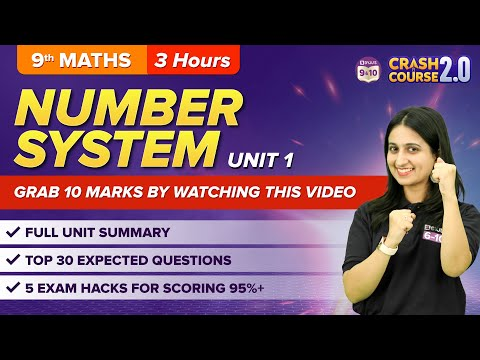Also Check:

## Number System Important Questions For Class 9 (Chapter 1)

Below given important Number system questions for 9th class students will help them to get acquainted with a wide variation of questions and thus, develop problem-solving skills.

Q.1: Find five rational numbers between 1 and 2.

Solution:

We have to find five rational numbers between 1 and 2.

So, let us write the numbers with denominator 5 + 1 = 6

Thus, 6/6 = 1, 12/6 = 2

From this, we can write the five rational numbers between 6/6 and 12/6 as:

7/6, 8/6, 9/6, 10/6, 11/6

Q.2: Find five rational numbers between 3/5 and 4/5.

Solution:

We have to find five rational numbers between 3/5 and 4/5.

So, let us write the given numbers by multiplying with 6/6, (here 6 = 5 + 1)

Now,

3/5 = (3/5) × (6/6) = 18/30

4/5 = (4/5) × (6/6) = 24/30

Thus, the required five rational numbers will be: 19/30, 20/30, 21/30, 22/30, 23/30

Q.3: Locate 3 on the number line.

Solution: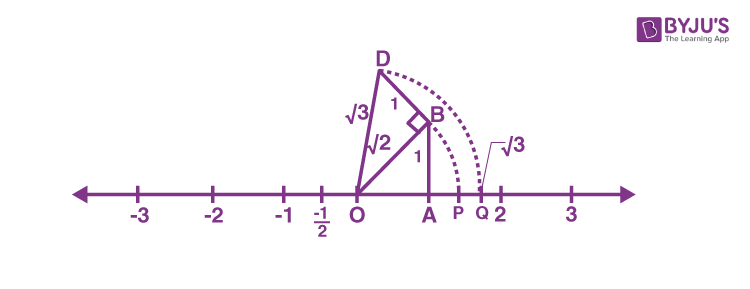Construct BD of unit length perpendicular to OB (here, OA = AB = 1 unit) as shown in the figure.

By Pythagoras theorem,

OD = √(2 + 1) = √3

Taking O as the centre and OD as radius, draw an arc which intersects the number line at the point Q using a compass.

Therefore, Q corresponds to the value of √3 on the number line.

Q.4: Are the square roots of all positive integers irrational? If not, give an example of the square root of a number that is a rational number.

Solution:

No, since the square root of a positive integer 16 is equal to 4. Here, 4 is a rational number.

Q.5: Find the decimal expansions of 10/3, 7/8 and 1/7.

Solution: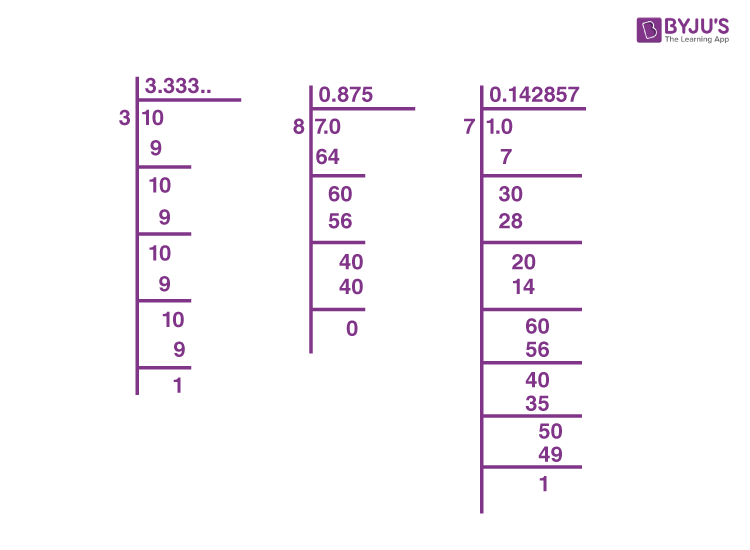Therefore, 10/3 = 3.3333…

7/8 = 0.875

1/7 = 0.1428571…

$$\begin{array}{l}\mathrm{Q.6: Show\ that\ 0.3333… = 0.\bar{3} \ can\ be\ expressed\ in\ the\ form\ p/q,\ where\ p\ and\ q\ are\ integers\ and\ q \neq 0.}\end{array}$$

Solution:

Let x = 0.3333….

Multiply with 10,

10x = 3.3333…

Now, 3.3333… = 3 + x (as we assumed x = 0.3333…)

Thus, 10x = 3 + x

10x – x = 3

9x = 3

x = 1/3

Therefore, 0.3333… = 1/3. Here, 1/3 is in the form of p/q and q ≠ 0.

Q.7: What can the maximum number of digits be in the repeating block of digits in the decimal expansion of 1/17? Perform the division to check your answer.

Solution: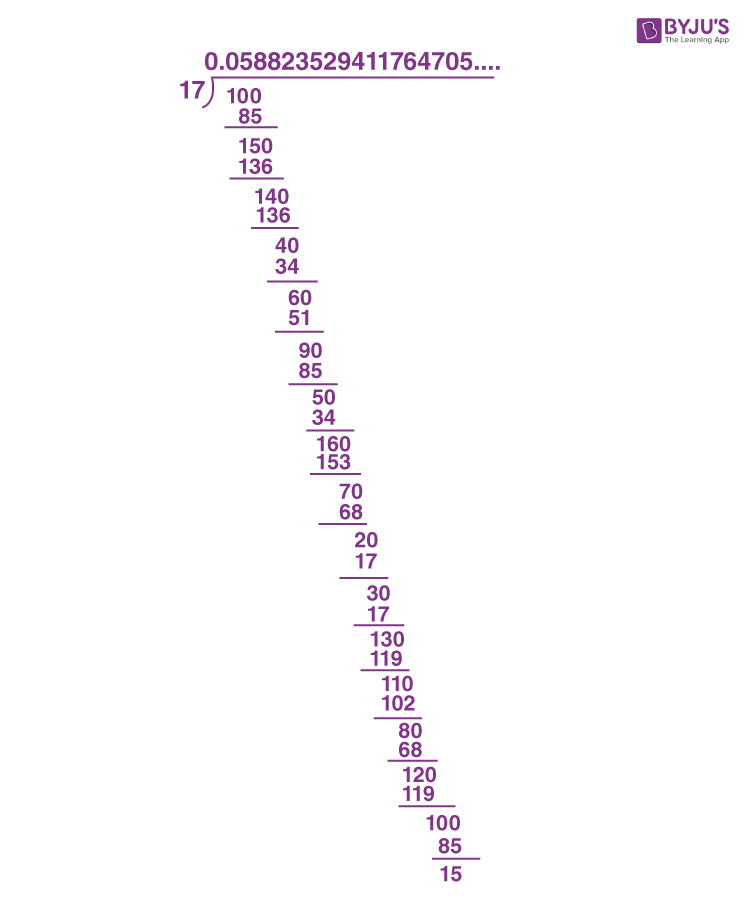Thus, 1/17 = 0.0588235294117647….

Therefore, 1/17 has 16 digits in the repeating block of digits in the decimal expansion.

Q.8: Find three different irrational numbers between the rational numbers 5/7 and 9/11.

Solution:

The given two rational numbers are 5/7 and 9/11.

5/7 = 0.714285714…..

9/11 = 0.81818181……

Hence, the three irrational numbers between 5/7 and 9/11 can be:

0.720720072000…

0.730730073000…

0.808008000…

Q.9: Visualise 3.765 on the number line, using successive magnification.

Solution:

Visualisation of 3.765 on the number line, using successive magnification is given below: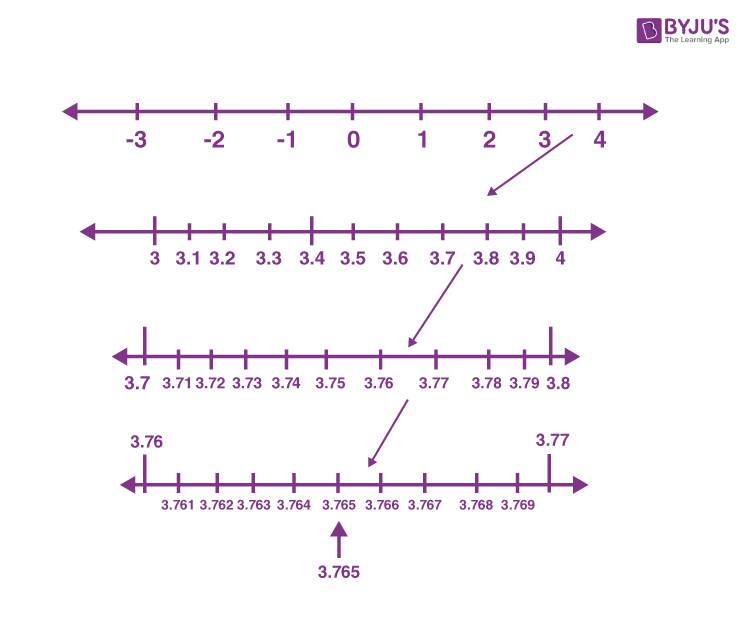Q.10: Add 22+ 53 and 2 – 33.

Solution:

(2√2 + 5√3) + (√2 – 3√3)

= 2√2 + 5√3 + √2 – 3√3

= (2 + 1)√2 + (5 – 3)√3

= 3√2 + 2√3

Q.11: Simplify: (√3+√7) (√3-√7).

Solution:

(√3 + √7)(√3 – √7)

Using the identity (a + b)(a – b) = a2 – b2,

(√3 + √7)(√3 – √7) = (√3)2 – (√7)2

= 3 – 7

= -4

Q.12: Rationalise the denominator of 1/[7+3√3].

Solution:

1/(7 + 3√3)

By rationalizing the denominator,

= [1/(7 + 3√3)] [(7 – 3√3)/(7 – 3√3)]

= (7 – 3√3)/[(7)2 – (3√3)2]

= (7 – 3√3)/(49 – 27)

= (7 – 3√3)/22

Q.13: Represent √(9.3) on the number line.

Solution:

Representation of √9.3 on the number line is given below: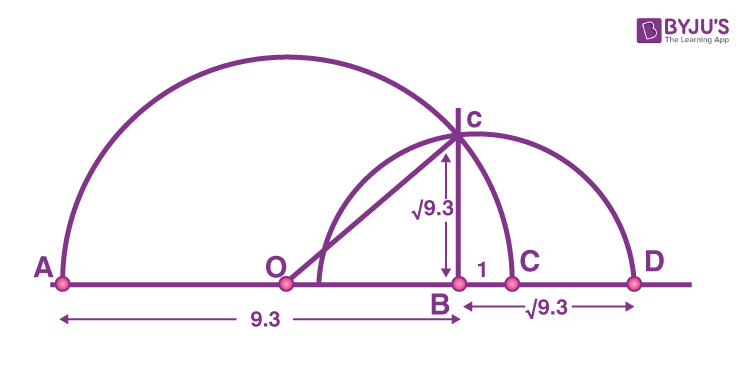Q.14: Simplify:

(i) 72/3.71/5
(ii) 101/2/101/4

Solution:

(i) 72/3.71/5

Bases are equal, so add the powers.

7(2/3 + 1/5)

= 7(10 + 3)/15

= 713/15

(ii) 101/2/101/4

Bases are equal, so subtract the powers.

= 10 (1/2 – 1/4)

= 101/4

Q.15:  What is the product of a rational and an irrational number?
a) Always an integer
b) Always a rational number
c) Always an irrational number
d) Sometimes rational and sometimes irrational

Explanation:

The product of a rational and an irrational number is always an irrational number.

For example, 2 is a rational number and √3 is irrational. Thus, 2√3 is always an irrational number.

Q.16: What is the value of (256)0.16 X (256)0.09?
a) 4
b) 16
c) 64
d) 256.25

(256)0.16 x (256)0.09 = (256)(0.16 + 0.09)

= (256)0.25

= (256)(25/100)

= (256)(1/4)

= (44)(1/4)

= 44(1/4)

= 4

Download BYJU’S-The Learning App and get personalised video content to understand the various concepts of Maths, defined for Class 9.

1. Sir,so much thanks for opening this learning app……its quiet much useful
For me….thanks a lot

• Thank you sir, these notes are very useful for exam

2. Hi, are there any solution for these questions? If yes please do share the link

• are there answers for the questions given above?

3. please give the solutions for the questions above. although it was very helpful. thank you.

4. Byjus is really very helpful for studies. It enables us to understand instead of craming. It is true that the teachers are Rockstars. Thank you Byjus.

6. where are the answers for the important questions???

7. Question of class 9 math chapter 1

9. Thx a lot,it is very helpful for me.

10. Pls upload exemplar questions like science

11. Sir please give the sample paper for class 9th best month I have to prepare for my exam please help me sir

12. sir please give solution for this

13. It is use full

14. Thank you so much.It is very useful for my exam.👍👍👍

16. Thanks it was so use full to us 😁😊😊 😊😁

17. its very useful to all

18. byjus is the best information provider for all age groups

19. It is a great app to be used by children for their studies. It helps me alot with my studies and I have even suggested my friends for the same application. Thanks for a great help. Thank you

20. it was very use full for us and it help me a lot at this examination time so, thank you

21. This is So Helpful 😄
Thnx to byjus

22. Best questions for workout thank you byjus

23. It was really helpful during examinations.
Because it helped me to understand the must questions of maths.

24. It was really useful for my term exam. Thank you so much byjus

25. It’s just the best app , which always have the best study things ever 👍☺️.
Keep it up !!